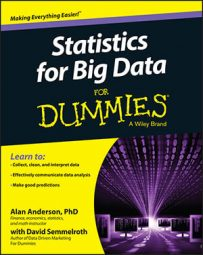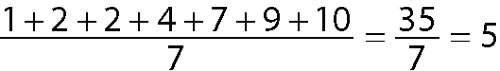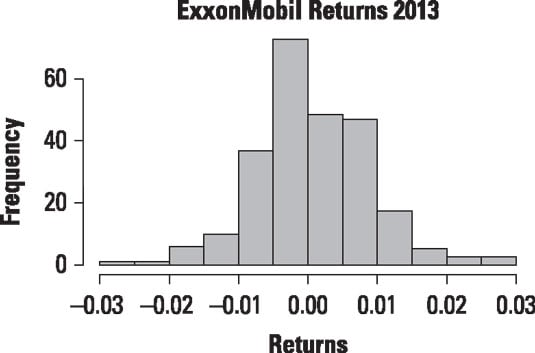##### Statistics for Big Data For DummiesYou identify the center of a dataset with several different summary measures. These include the big three: mean, median, and mode. You calculate the mean of a dataset by adding up the values of all the elements and dividing by the total number of elements. For example, suppose a small dataset consists of the number of days required to receive a package by the residents of an apartment complex:

1, 2, 2, 4, 7, 9, 10

The mean of this dataset would be the following:The average length of time for the residents to receive a package is 5 days.

The median of a dataset is a value that divides the data in half. The first half contains the smallest elements and the second half consists of the largest elements. In the previous example, because the data consist of seven observations, the fourth smallest value would be the median:

1, 2, 2, 4, 7, 9, 10

The median is 4, because half of the observations are less than 4, and half are greater than 4.

The mode of a dataset is simply the most frequently occurring value. With the package delivery example, the mode is 2.

For a real-world example, this figure shows a histogram for daily returns to ExxonMobil stock in 2013.Histogram of daily returns to ExxonMobil stock for 2013.

Each bar represents a range of values; the width of each interval is 0.005. The heights of the bars indicate how many returns fell within each interval. The histogram makes it easy to see which ranges of values occurred the most frequently and which occurred the most infrequently.

The histogram shows that most of the returns are close to the mean, which is 0.000632 (0.0632 percent). The median is −0.000118, and the mode could be considered to be the range of values between −0.005 and 0.